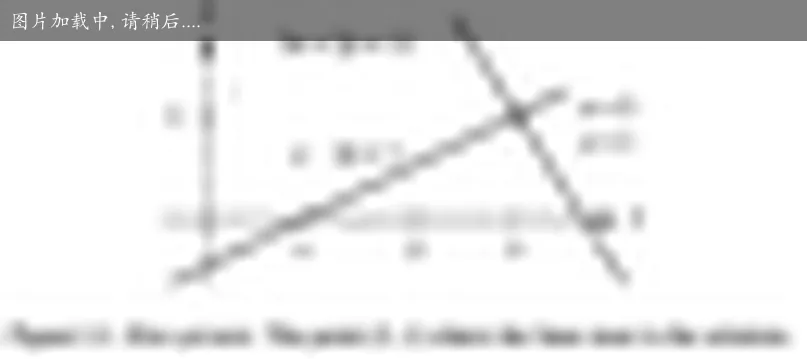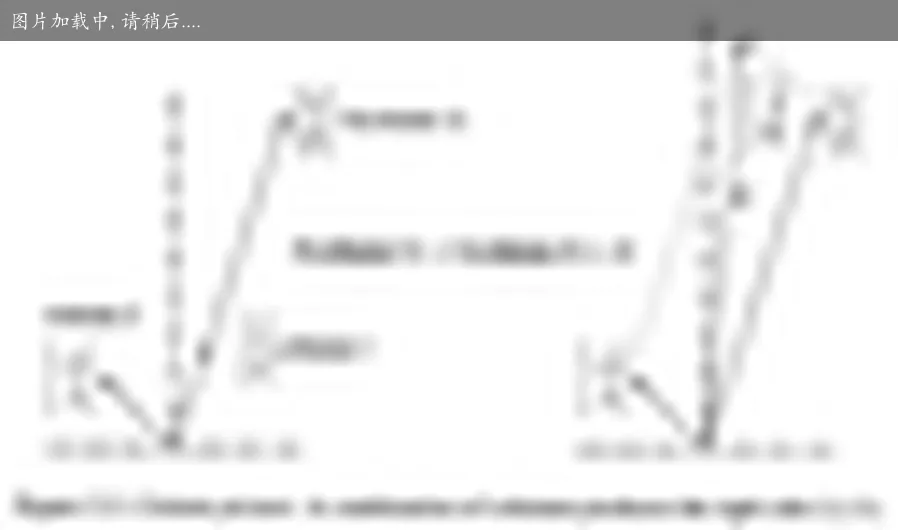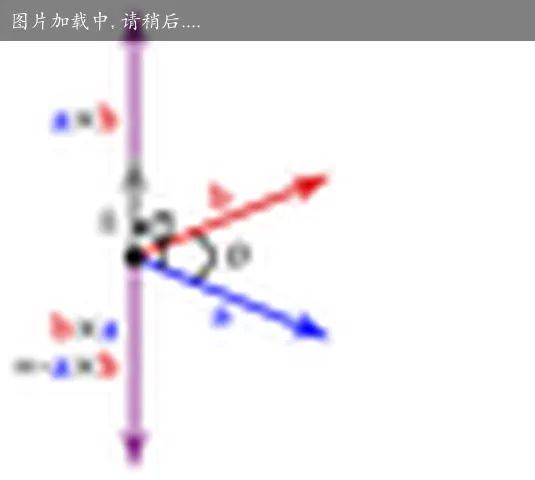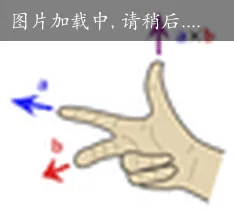template<typename T>
Matrix4x4<T> perspective(T fovy, T aspect, T near, T far){

T q = 1.0f / tan((0.5f * fovy) * (3.14 / 180));
T A = q / aspect;
T B = (near + far) / (near - far);
T C = (2.0f * near * far) / (near - far);

return Matrix4x4<T>(
Vector4<T>(A,0,0,0),
Vector4<T>(0,q,0,0),
Vector4<T>(0,0,B,-1),
Vector4<T>(0,0,C,0));
}


## 01 课

### 从字面理解什么是线性代数

\left\{ \begin{alignedat}{2} &x \space- \space&2&y \space=\space 1 \\ 3&x \space+ \space&2&y \space=\space 11 \end{alignedat} \right.### 内积与向量的方向

$a=\left[a_{1}, a_{2}, \ldots a_{n}\right]$ $b=\left[b_{1}, b_{2}, \ldots b_{n}\right]$ $a \bullet b=a_{1} b_{1}+a_{2} b_{2}+\ldots+a_{\mathrm{n}} b_{n}$

#### 几何意义

$a \bullet b=|a| \ |b| \cos \theta$

### 叉乘

$\begin{array}{l} a=\left(x_{1}, y_{1}, z_{1}\right) \\ b=\left(x_{2}, y_{2}, z_{2}\right) \end{array}$ $a \times b=\left(y_{1} z_{2}-y_{2} z_{1},-\left(x_{1} z_{2}-x_{2} z_{1}\right), x_{1} y_{2}-x_{2} y_{1}\right)$

#### 几何意义$| \vec a \times \vec b | = |\vec a| \cdot |\vec b| \cdot \sin \theta$